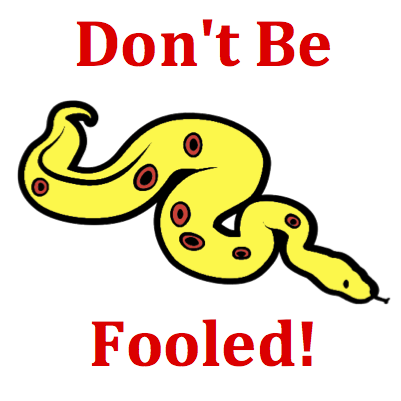# Kinematic Graphing - Mission KG6 Detailed HelpThe motion of an object is depicted by a velocity-time graph. Various time intervals during the motion are depicted by a letter. During which time interval(s) is the object moving with slowing down (getting slower)? List all that apply ... .Velocity-Time Graphs: Velocity versus time graphs represent changes that occur in an object's velocity with respect to time. The slope of the line is the acceleration (change in velocity divided by the change in time) of the object. The area under the line (between the line on the graph and the time axis) is the displacement of the object.Many students become confused by the phrase slowing down. Because of the presence of down in the phrase, they begin to restrict their answers to those lines which are sloping down. But don't be fooled! Sloping down does not mean slowing down. A line that simply slopes down is a line with a negative slope and thus a negative acceleration. An object which slows down is an object with a decreasing speed. An object can move in the positive direction (and have a line sloping upward) and still be slowing down (if the line is approaching the time axis). See Think About It section.Many questions in this mission can be answered with a mixture of good math sense and logical thinking. The graph is a plot of velocity. Velocity is a speed with a direction. Since the magnitude of the velocity is the speed, slowing down means getting smaller velocity values. The horizontal axis on the graph represents a 0 velocity. As a line on the graph approaches this 0 value, the velocity value is decreasing. Whether the line starts below the axis and goes up and towards it or starts above the axis and goes down and towards it, the velocity values are getting smaller.